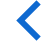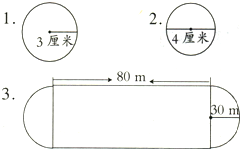##圆的面积公式

S=πr21：解：r=3; r2=9;

S=3.14 x 32=28.26（cm2

2：解：d=4cm；r=2cm；

S=3.14 x 22=12.56（cm2

3：解：左右两边的半圆可以拼成一个圆。

S=3.14 x 302=2826（m2

S长方形=80 x (30 x 2)=4800（m2

S=S长方形+S=2826+4800=7626（m2

• 球体的体积公式
• 球体的表面积公式
• 圆的面积公式
• 圆的周长公式